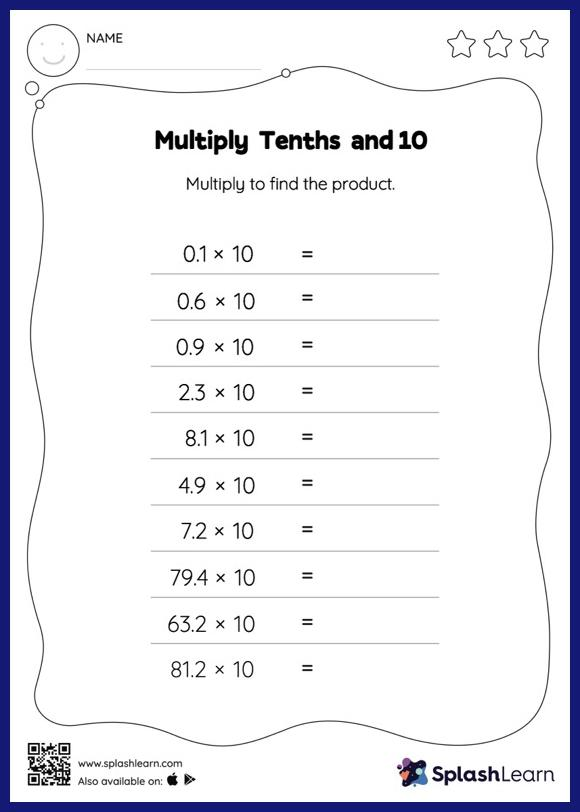# Multiply Tenths and 10: Horizontal Multiplication Worksheet

Home > Multiply Tenths and 10: Horizontal MultiplicationChallenge your young math wizards to solve this multiply tenths and 10 worksheet. When a decimal is multiplied by 10, the decimal point moves one place to the right. Students use this understanding to multiply tenths and 10 in this worksheet. In this worksheet, students practice solving problems written in the horizontal format. How numbers are laid out in a problem affects the method a student employs to solve it. Therefore to develop actual fluency and mastery of multiple strategies, students must practice different formats.